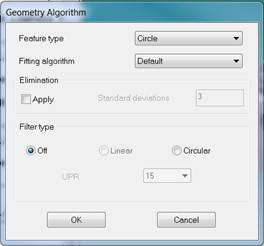# Filtering options available when scanning circles

The following shows the default dialog box shown from Settings > Geometry Algorithm after Circle is selected from Feature type:### Algorithms available

#### Default

The default is Least Squares.

#### Least Squares

This minimises the average squared error. This algorithm uses Gaussian elimination.

#### Maximum Inscribed

The biggest circle that fits inside all the points. The algorithm used is Tschebyscheff.

#### Minimum Inscribed

The smallest circle that encloses all the points. The algorithm used is Tschebyscheff.

#### Minmax

The circle is fitted halfway between two circles, with a common centre, which have the minimum distance between them which encloses all the data. This has the effect of minimising the maximum deviation of any data point from the fitted circle. The two circles are not the same as those produced using Maximum inscribed and Minimum inscribed, due to their common centres. The algorithm used is Tschebyscheff.

### Elimination

Use the Elimination box to set up the standard deviation algorithm used to apply a filter to the data. This means that you can reduce noise and achieve a good approximation of the surface.

#### Apply

Applies the standard deviation filter to the data from circles. This adds ELIMINATE to the GEOALG command of your program.

#### Standard Deviations

This is the number of standard deviations above which points are considered to be noise. Above this, points are filtered out.

### Filter Type

#### Off

Nothing is applied.

#### Circular

Applied to circular features. The more points obtained when measuring the feature, the better approximation. There is a minimum requirement for points taken, to be 10 times the number of undulations required in the circumference. As per DMIS specification, a minimum of 10 points per degree are required for roundness.

#### UPR

UPR stands for undulations per revolution; it sets the number of peaks allowed around the circumference. If you were to use 5, the 5 most significant peaks are calculated and the rest are filtered out. As a rough rule, a bigger circle would use a higher UPR value. The choice of UPR is also dependent upon the finish condition of a component, so a rough casting might be filtered at 15 UPR, whereas a polished, finished part might be 500. These finishes will have different effects on the form.

### Applications

The following example code shows a circle measured at 0, 0, -4.309 in the XY plane, using Least Squares method. It is then outputted using this algorithm, Maximum Inscribed and Minimum Circumscribed. Data over 3 standard deviations away from the mean was omitted.

T(Example_Tolerance)=TOL/DIAM,-0.1,0.1

GEOALG/CIRCLE,LSTSQR,ELIMINATE,STDDEV_LIMIT,3,FILTER,OFF

P(PArc1)=PATH/ARC,CART,0,0,-4.309401,0,0,1,40,0,360,1,0,0
MODE/PROG,MAN
F(Measured_Circle_Least_Square_Default)=FEAT/CIRCLE,INNER,CART,0,0,-2.309,0,0,1,80
MEAS/CIRCLE,F(Measured_Circle_Least_Square_Default),7
PAMEAS/DISTANCE,0.5,SCNVEL,MMPS,100,P(PArc1),-1,0,0
ENDMES

OUTPUT/FA(Measured_Circle_Least_Square_Default),TA(Example_Tolerance)

GEOALG/CIRCLE,MAXINS,ELIMINATE,STDDEV_LIMIT,3,FILTER,OFF

F(Measured_Circle_Max_Inscribed)=FEAT/CIRCLE,INNER,CART,0,0,-2.309,0,0,1,80
CONST/CIRCLE,F(Measured_Circle_Max_Inscribed),TR,FA(Measured_Circle_Least_Square_Default)

OUTPUT/FA(Measured_Circle_Max_Inscribed),TA(Example_Tolerance)

GEOALG/CIRCLE,MINCIR,ELIMINATE,STDDEV_LIMIT,3,FILTER,OFF

F(Measured_Circle_Min_Inscribed)=FEAT/CIRCLE,INNER,CART,0,0,-2.309,0,0,1,80
CONST/CIRCLE,F(Measured_Circle_Min_Inscribed),TR,FA(Measured_Circle_Least_Square_Default)

OUTPUT/FA(Measured_Circle_Min_Inscribed),TA(Example_Tolerance)

NOTE: If neither elimination nor filter are used when the algorithm is changed, an EVAL line will need to be included between feature re-fitting and OUTPUT. If elimination or a filter is applied, the copy causes a re-evaluation as well as the new fitting.